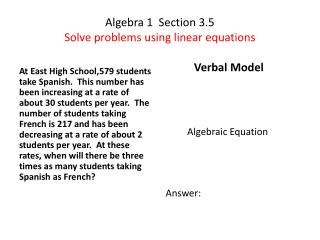# Algebra 1 Section 3.5 Solve problems using linear equations - PowerPoint PPT PresentationDownload PresentationAlgebra 1 Section 3.5 Solve problems using linear equations

Algebra 1 Section 3.5 Solve problems using linear equationsDownload Presentation## Algebra 1 Section 3.5 Solve problems using linear equations

- - - - - - - - - - - - - - - - - - - - - - - - - - - E N D - - - - - - - - - - - - - - - - - - - - - - - - - - -
##### Presentation Transcript

1. Algebra 1 Section 3.5Solve problems using linear equations At East High School,579 students take Spanish. This number has been increasing at a rate of about 30 students per year. The number of students taking French is 217 and has been decreasing at a rate of about 2 students per year. At these rates, when will there be three times as many students taking Spanish as French? Verbal Model Algebraic Equation Answer:

2. At North High School, 176 students buy non-spiral notebooks from the school store. This number has been increasing at a rate of about 24 per year. The number of students buying spiral notebook is 331 and has been decreasing at a rate of about 15 per year. At these rates, when will the number of spiral notebooks sold be half the number of non-spiral notebooks sold? Verbal Model Algebraic equation Answer:

3. Tim walks east from point A at a speed of 6 km/h. June walks west from point B at a rate of 8 km/h. If point A and point B are 35 km apart, when will they meet? Verbal Model Algebraic equation Answer:

4. Jim and Mike start at the same point. Jim travels east at 60 km/h. Mike starts 2 hours later and travels east at 90 km/h. When will Mike catch up to Jim? Verbal model Algebraic Equation Answer: